### IMO Shortlist 2012 problem N5

Kvaliteta:
Avg: 0,0
Težina:
Avg: 7,5
For a nonnegative integer$n$ define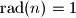$\text{rad}(n)=1$ if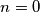$n=0$ or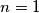$n=1$, and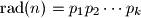$\text{rad}(n)=p_1p_2\cdots p_k$ where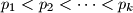$p_1 are all prime factors of$n$. Find all polynomials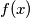$f(x)$ with nonnegative integer coefficients such that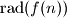$\text{rad}(f(n))$ divides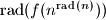$\text{rad}(f(n^{\text{rad}(n)}))$ for every nonnegative integer$n$.
Izvor: Međunarodna matematička olimpijada, shortlist 2012